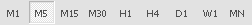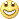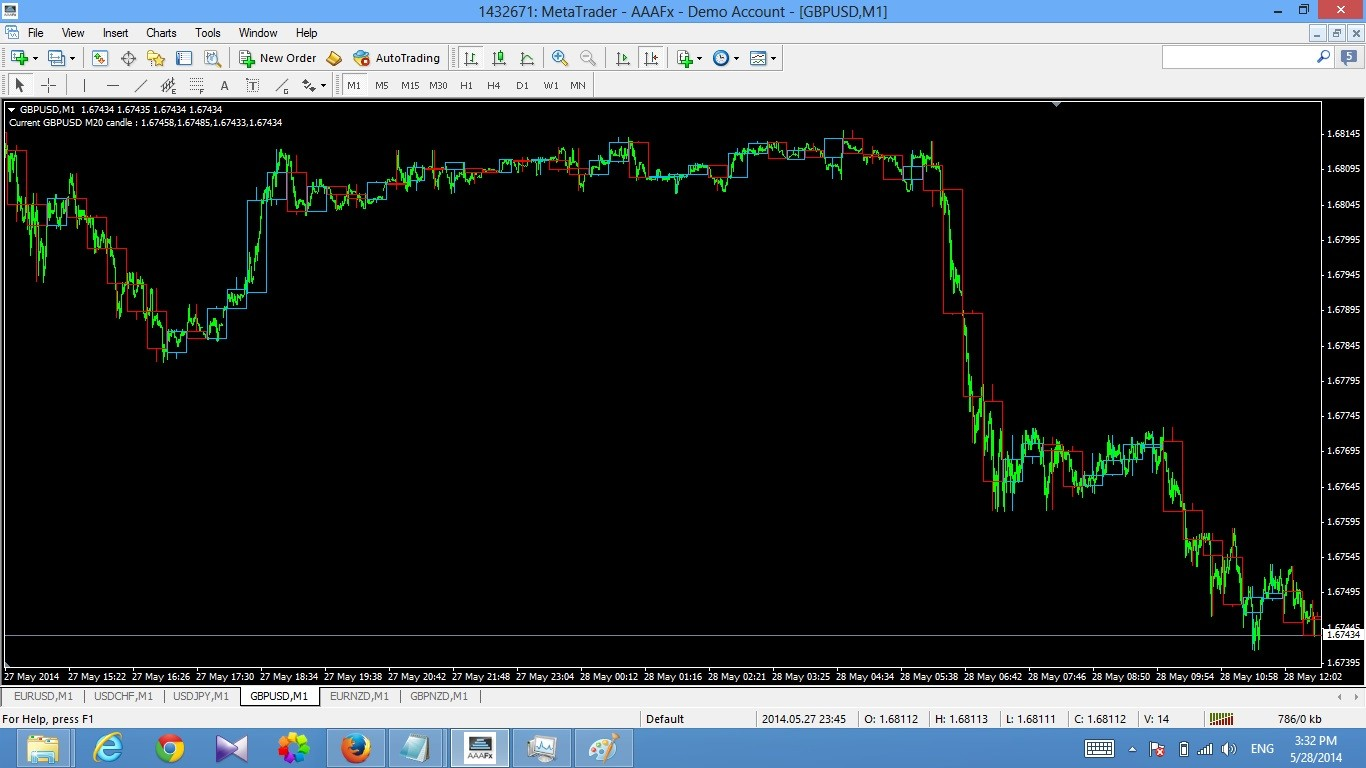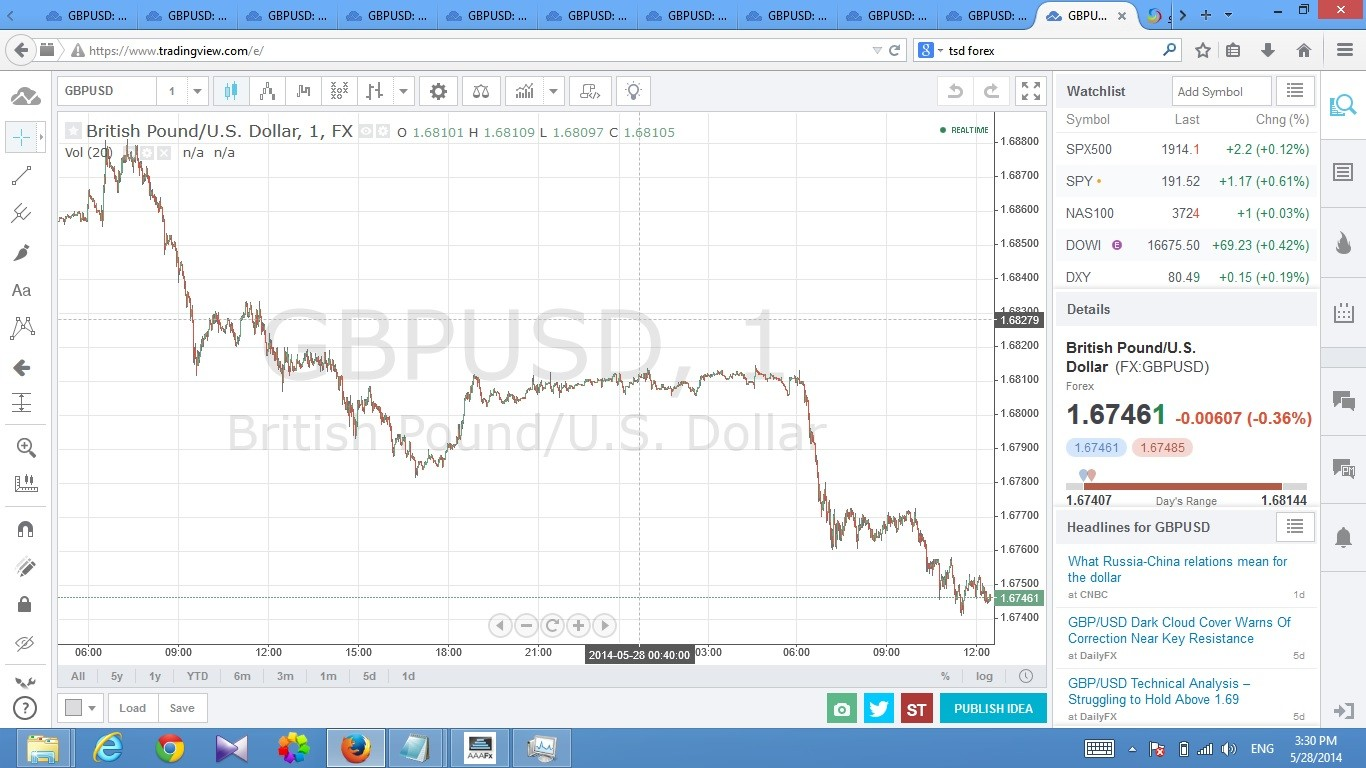# Multi Timeframe Indicators - page 7209493

justin7:
that's the same thing. Can that be made to show multi time frame? I want to try this out with a 5m+15m combination

Justin7, it already is multi time frame, this is it on m5 and m15.

Files:165

Hi Pipscalp

1 . GO AVANCED

2. You will taken to new page

4. Click on Attachment button on top right, new window will open

6. New window , Click Select Files

9. Done

Try It146

mrtools:
Justin7, it already is multi time frame, this is it on m5 and m15.

when i load it on a chart it only shows the current time frame how do i load a second one with the next higest time frame :/? sorry i'm a noob at programming154514

justin7:
when i load it on a chart it only shows the current time frame how do i load a second one with the next higest time frame :/? sorry i'm a noob at programming

justin7

Set the TimeFrame parameter to the desired value (you can use the values from the periodicity bar instead of entering the number of minutes : m1,m5,m15,m30,h1,h4,d1,w1 and mnFiles:154514

MACD cross zero separate (originally posted here https://www.mql5.com/en/forum/173574/page260 ) updated for new metatrader 4 : macd_cross_zero_separate_mtf_nmc.mq4

Files:52

mrtools:

Thanks mrtools52

hi my dera mladen i have proplem in mt4 not give me view like trading viewthis from mt4 after full zoomthis from tradingview.com give me all the trend

i want mt4 give me view like one from trading view

Files:
pic2.jpg 200 kb
pic1.jpg 259 kb52

but i need the indicator below for mt4

study(title="Stochastic RSI", shorttitle="Stoch RSI")

source = close

lengthRSI = input(14, minval=1), lengthStoch = input(14, minval=1)

smoothK = input(3, minval=1), smoothD = input(3, minval=1)

rsi1 = rsi(source, lengthRSI)

k = sma(stoch(rsi1, rsi1, rsi1, lengthStoch), smoothK)

d = sma(k, smoothD)

plot(k, color=blue)

plot(d, color=orange)

h0 = hline(80)

h1 = hline(20)

fill(h0, h1, color=purple, transp=80)154514

mikocairo55:

but i need the indicator below for mt4

study(title="Stochastic RSI", shorttitle="Stoch RSI")

source = close

lengthRSI = input(14, minval=1), lengthStoch = input(14, minval=1)

smoothK = input(3, minval=1), smoothD = input(3, minval=1)

rsi1 = rsi(source, lengthRSI)

k = sma(stoch(rsi1, rsi1, rsi1, lengthStoch), smoothK)

d = sma(k, smoothD)

plot(k, color=blue)

plot(d, color=orange)

h0 = hline(80)

h1 = hline(20)

fill(h0, h1, color=purple, transp=80)

mikocairo55

As far as I see they are the same

The indicator from your post is a sma smoothed stochastic with an addition of a signals line - the same thing is done in the stochastic RSI. If you set the same parameters you are going to get same values165